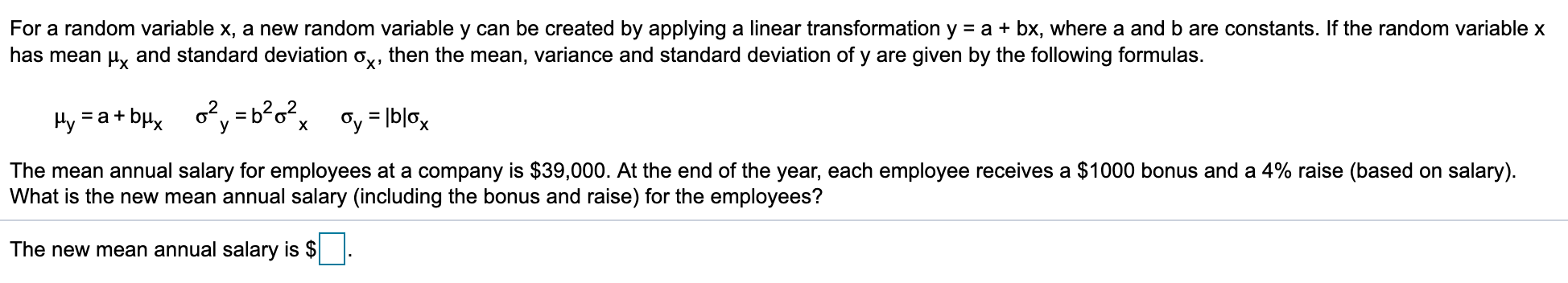# For a random variable x, a new random variable y can be created by applying a linear transformation y a bx, where a and b are constants. If the random variable xhas mean and standard deviation o,, then the mean, variance and standard deviation of y are given by the following formulas.-a+b 2y=b?o2xGy bloxThe mean annual salary for employees at a company is \$39,000. At the end of the year, each employee receives a \$1000 bonus and a 4% raise (based on salary).What is the new mean annual salary (including the bonus and raise) for the employees?The new mean annual salary is \$

Question

I have no idea how to solve this. This was not covered at all in my text.help_outlineImage TranscriptioncloseFor a random variable x, a new random variable y can be created by applying a linear transformation y a bx, where a and b are constants. If the random variable x has mean and standard deviation o,, then the mean, variance and standard deviation of y are given by the following formulas. -a+b 2y=b?o2x Gy blox The mean annual salary for employees at a company is \$39,000. At the end of the year, each employee receives a \$1000 bonus and a 4% raise (based on salary). What is the new mean annual salary (including the bonus and raise) for the employees? The new mean annual salary is \$ fullscreen
check_circleExpert Solution
Step 1

From the given information, the mean annual salary for employees at a company is \$39,000, each employee receives a \$1000 bonus at end of the year and there is 4% raise based on salary.

Let us define the random variable X as the annual salary of employees with mean salary µx=39,000 and there is a ...

### Want to see the full answer?

See Solution

#### Want to see this answer and more?

Solutions are written by subject experts who are available 24/7. Questions are typically answered within 1 hour*

See Solution
*Response times may vary by subject and question
Tagged in

### Statistics